# Wandering point

(diff) ← Older revision | Latest revision (diff) | Newer revision → (diff)
A pointin the phase spaceof a dynamical system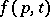with a neighbourhood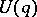for which there exists a moment in timesuch that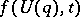has no common points withfor all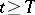(all points of, from some moment on, leave the neighbourhood). A pointwithout such a neighbourhood is said to be non-wandering. This property of a point — to be wandering or non-wandering — is two-sided: Ifhas no common points with, thenhas no common points with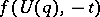. A wandering point may become non-wandering if the spaceis extended. For instance, ifis a circle with one rest point, all points of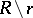are wandering points. They become non-wandering if the points of some spiral without rest points, winding itself around this circle from the outside or from the inside, are added to.
A set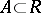is positively recursive with respect to a setif for allthere is a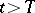such that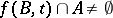. Negatively recursive is defined analogously. A pointis then non-wandering if every neighbourhood of it is positively recursive with respect to itself (self-positively recursive). A pointis positively Poisson stable (negatively Poisson stable) if every neighbourhood of it is positively recursive (negatively recursive) with respect to. A point is Poisson stable if it is both positively and negatively Poisson stable. If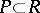is such that every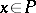is positively or negatively Poisson stable, then all points ofare non-wandering. See also Wandering set.